# A preliminary on generative modeling using neural networks

Published:

## Introduction

Deep neural networks have found their way to wide variety of applications but only recently has the application of these networks for unsupervised and semi supervised learning leveraging large unlabeled data has been worked upon.

Generative modeling is a branch of machine learning that attempts at modeling the probability distribution of high dimensional data, for example - images. Images present themselves as high dimensional data points that are mostly correlated. Take for instance a character image specifically say digits 0-9. It is highly unlikely that given the left half of the image contains the left half of 0; the right half would contain the left half of say 5. The generative models job is to capture these kinds of dependencies between pixels. In case of images by training a model to generate images as real as possible or as close to the actual dataset as possible is a good way to capture these dependencies.

### Hidden/ Latent Variables

Intuitively say we have a vector of latent variable z and assuming we can find a function f(z, θ) where θ is a vector of parameters. Now, training a generative model is to define this function such that for every data point X in our dataset, there is one setting of the latent variables which is able to generate something very similar to X. By being able to obtain such a function we have found the underlying/ defining low dimension space – which can be potentially used for various other tasks like classification, clustering and such.

### Auto Encoders

Auto Encoder is a methodology which tries to learn a function f(X| θ) = X, i.e an identity mapping. Seems like a trivial task, but by forcing specific constrains such as compressing the input from high dimension to lower dimension and then reconstructing we can discover interesting structure in data if one exists. In fact a simple auto encoder can learn features similar to a principal component analysis. Auto encoders have recently received attention with the advent of neural networks that have proved to be very good function approximators and their ability to encode information efficiently. Training auto encoders is theoretically nothing but a maximum likelihood technique to learn a function. Several improvements to the basic auto encoder are being studied and applied. These improvements are primarily enforced by adding additional constrains like sparsity on the latent dimension space (Sparsity auto encoders) or by defining different loss function to train the network on for e.g. by using Kullback Leibler divergence term in the loss we can train a network with strong distribution prior on the latent variables (Variational Auto encoder).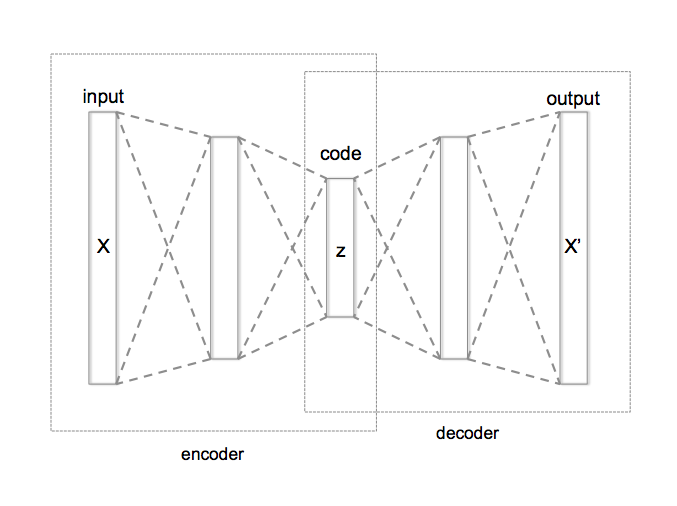Source: Wikipedia

Generative adversarial networks are methods that are based on game theory. The idea is to have two networks

•  Generator network G(z θ) that produces samples from the data distribution by transforming a noisy vector z.
• Discriminator network D(X) that tries to differentiate a real data point from that which was generated.

By jointly training these two networks to play a cat and mouse game we hope to achieve a representation that is useful to describe the dataset. GAN are very unstable to train and so require careful selection of model activations and the model itself. The problem is mainly due to the fact that the optimization techniques used for training these networks are not meant for finding Nash equilibrium which is the ideal point where we want the networks to be at after training.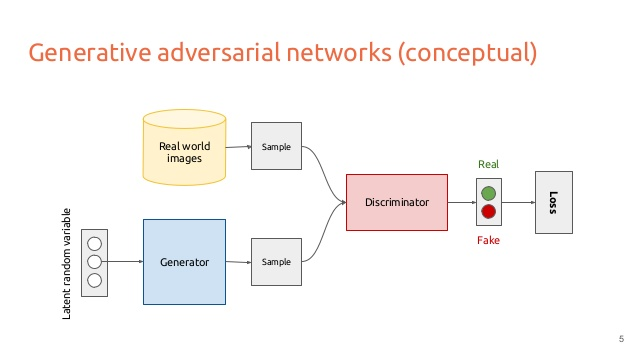Source: Slideshare.net

## Experiments

Simple AutoEncoder

The figure below presents results obtained on MNIST using a simple auto encoder for the purpose of visualization with L2 loss between generated image and the actual image as the supervisory signal. The model is made up of 6 fully connected layers with a latent variable dimension of 3. It is interesting to notice how the network has separated the digits and formed clusters.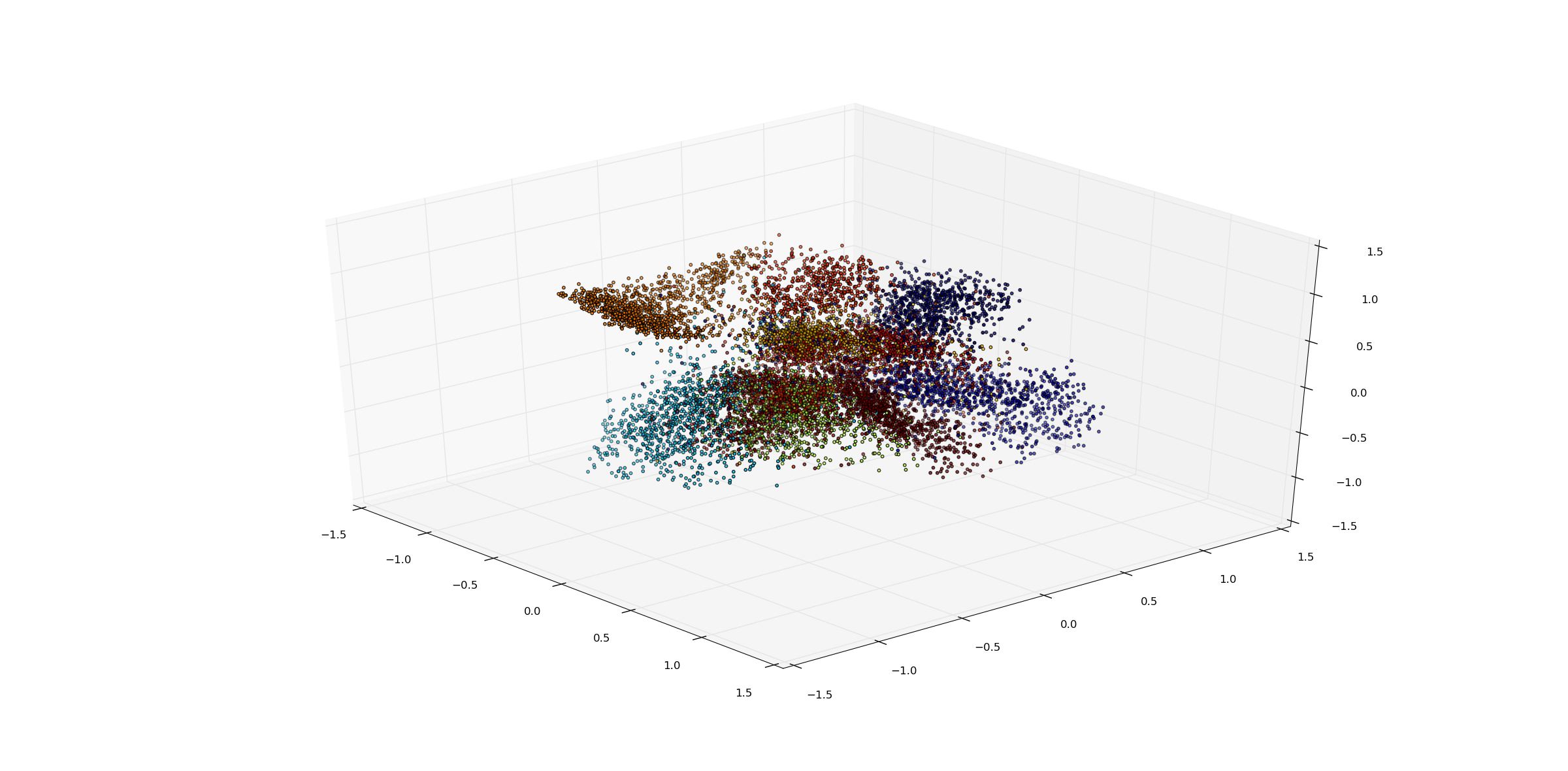Variational Auto Encoder

Variational Auto Encoders differ from other autoendoer in that they have strong probablistic inperpretation and priors on the latent variable space and are significantly faster compared to the simple autoencoder. The figure below presents the result of VAE on MNIST data. The network encoder is made up of 4 fully connected layers with the first two layers shared for the mean and log variance encoder layers. The decoder network is made up of 3 layers.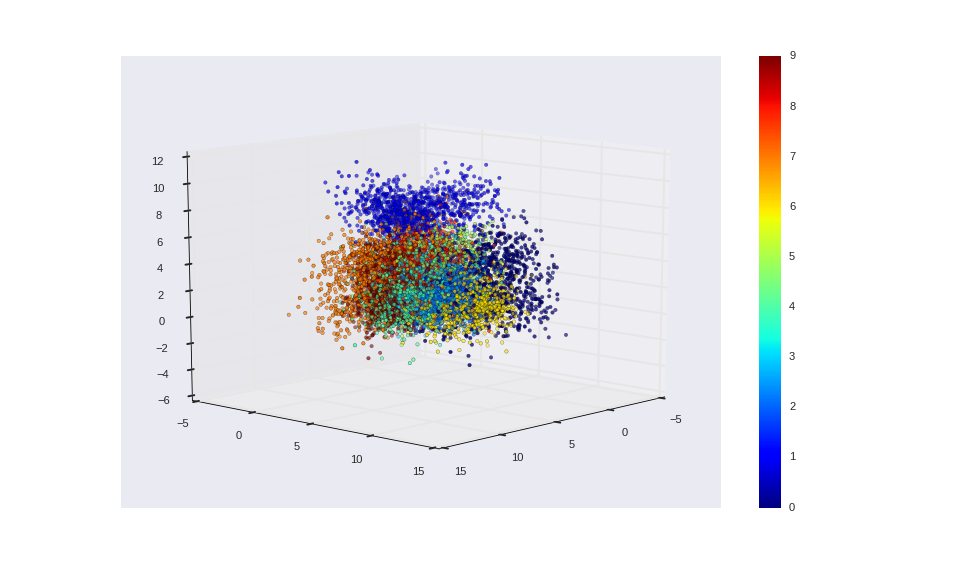Observations on training VAE:

• The loss objective in VAE is the sum of reconstruction loss and the KL divergence - this presents itself as a problem since during training reconstruction loss dominates the process. Trying to combine these losses in a weighted sum introduces a hyperparameter in the model. Also as was pointed by someone this would mean that we are no longer optimizing standard lower variance.
• The log variance activations tend to converge to zero even though from the above one wouldn’t have expected this - Overfitting? So turns out this is expected as the KL divergence term encourages this very behavior as I expected. Another problem that was pointed out was a shortcoming in my implementation - ReLuing the mu and log var activation, an unnecessary constrain on mu and variance. Fixed implementaion results are as seen in the second graph.
• The KL divergence is strictly positive but at times the training end up with a negative KL divergence loss. There should be a way to enforce this constrain in the network?! By the nature of optimization itself the non negativity of KL divergence, a negative value basically means there is a mistake on the training objective.

Sample generated images: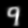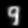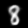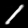Activations on mean and log variance encoder: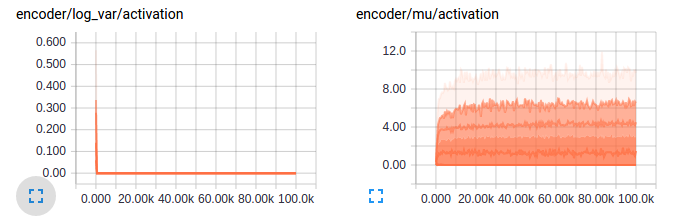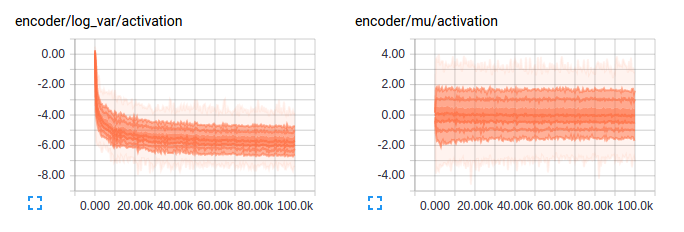GANs are such a pain to train in that they are very unstable and the training requires careful tuning of parameters - will mostly create separate notes on training GAN and it’s results. And I did - notes can be found here: link

Code for experiments available at: TensorflowProjects/Unsupervised_Learning

Logs for the purpose of visualization using tensorboard can be found in logs folder

References:

1. Tutorial on Variational Auto Encoder by Carl Doersch
2. Generative Adversarial Nets by Goodfellow et.al.
3. Unsupervised representation learning with deep convolutional generative adversarial networks by Radford et.al.

Tags:

Categories: